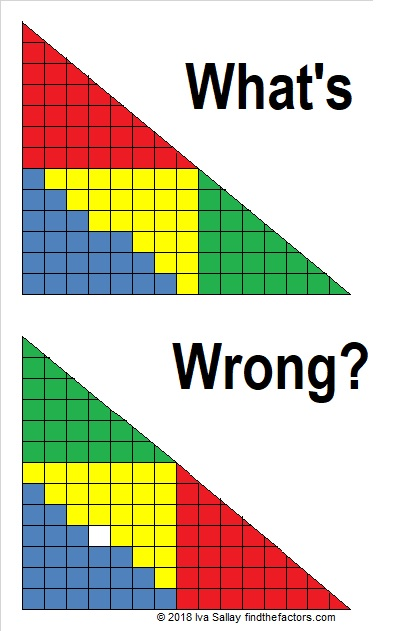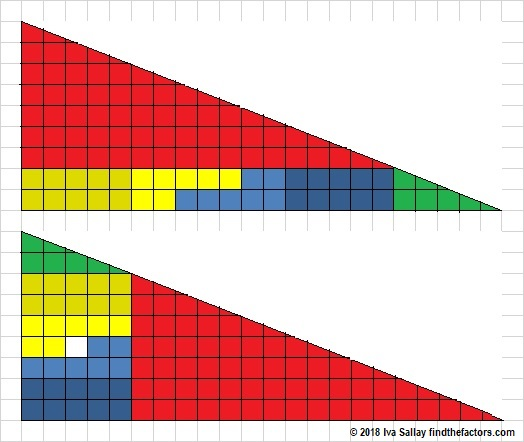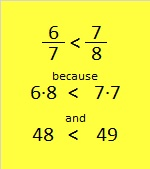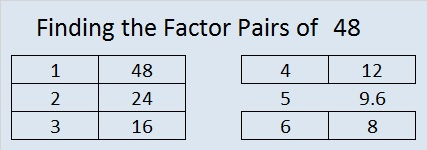# 48 and What’s wrong with this?

Here is a four piece puzzle arranged two different ways. There are 24 blue squares and 24 yellow squares. 24 + 24 = 48. Read on to see why the factors of 48 are significant to this puzzle.Those triangles are some fabulous mathematical slight of hand.  Something is wrong with the puzzle, but what?

Something is fishy with these two triangle puzzles, too:And with these two triangles made with six puzzle pieces!If I had time, I could make an infinite number of these types of puzzles. Why do we get a solid triangle sometimes, but one with a hole in it the other times?

Believe it or not, the answer to the triangle puzzles has something to do with factoring.

Here’s that first puzzle again, but smaller:The first triangle doesn’t have a hole it while the second one does becauseWho would have thought that comparing fractions could turn into a type of magic trick?!!

Both triangles have 48 blue or yellow squares, but the second triangle wants you to think it should have 49.

(Psst….6/7 is also the slope of the green triangle and 7/8 is the slope of the red triangle. Since the two triangles don’t have identical slopes, the big “triangles” formed in the puzzles aren’t really triangles at all.)

I made all the puzzles in this post by comparing fractions. In each case, I simply used two consecutive composite numbers and then factored them and came up with the fractions I wanted to use. The numerators and the denominators of the fractions became the sides of the triangles.

Now, what do you think of this video of a never-ending chocolate bar?

Let me tell you a little bit about the number 48:

48 is a composite number. 48 = 1 x 48, 2 x 24, 3 x 16, 4 x 12, or 6 x 8. Factors of 48: 1, 2, 3, 4, 6, 8, 12, 16, 24, 48. Prime factorization: 48 = 2 x 2 x 2 x 2 x 3, which can also be written 48 = 2⁴ x 3.Sometimes 48 is a clue in the FIND THE FACTORS puzzles. Even though it has many other factors, we use only 6 x 8 for the FIND THE FACTORS 1-10 puzzles and 6 x 8 or 4 x 12 for the FIND THE FACTORS 1-12 puzzles.## 2 thoughts on “48 and What’s wrong with this?”

1.fluffy

This is an old chestnut! Of course, if you were to look at the slope of the blue triangle (2/5) and the red triangle (3/8) you’d see that they’re almost, but not quite, equal; the figure on the bottom has a little bit more area than the one on the top.

2.ivasallay

Great observations!

This site uses Akismet to reduce spam. Learn how your comment data is processed.